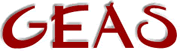[ < ] [ > ] [ << ] [ Up ] [ >> ] [Top] [Contents] [Index] [ ? ]

### 2.3.10 Array functions

Arrays are actually not arrays, but rather ordered lists of LPC data types. They can be made to contain any type of data, including other arrays. Keep in mind that arrays unlike other data types are copied by reference rather than by value. This means that when you assign an array to variable you do not copy the array, you merely store a reference, a pointer to the array, in the variable.

 ```e.g. string *arr1, *arr2; arr1 = ({ 1, 2, 3 }); arr2 = arr1; arr2 = 5; dump_array(arr1); /* * The output is: * * (Array) *  = (int) 1 *  = (int) 5 *  = (int) 3 */ ```

So, as you can see, changing the array `arr2' effectively changes the contents of `arr1' as well. You need to make a copy of `arr1' first, to make it unique. For example by simply adding an empty array `({})' to it.

As you have learnt arrays can be automatically allocated simply by writing them in the code, by adding elements to them or adding arrays to each other. However, if you need to allocate an array immediately to a specified size, you can use the `allocate()` efun. It takes as an argument the size of the array you want and initializes all elements, regardless of array type, to 0.

 ```mixed *allocate(int num) e.g. string *str_arr; str_arr = allocate(3); str_arr = "Fatty is a flabby blimp"; dump_array(str_arr); /* The result is: (Array)  = (int) 0  = (string) "Fatty is a flabby blimp"  = (int) 0 */ ```

If you need to find out if a particular item is a member of an array or the index of that item, you use the efun `member_array()`. It takes as arguments an array and an item of any type, returning the index if it is part of the array and -1 if it isn't. If several instances of the searched for item exists in the array, the first index is returned.

 ```int member_array(mixed arr, mixed elem) e.g. int *arr = ({ 1, 55443, 123, -3, 5, 828, 120398, 5, 12 }); int index; // Replace all instances of the value '5' with '33' while ((index = member_array(arr, 5)) >= 0) arr[index] = 33; ```

A very important efun to use with arrays is `sizeof()`. It simply returns the size, the number of elements, in an array. It's very common that you need to loop through all elements of an array to do something, or perhaps just find the last element, and then you need to know the size.

 ```int sizeof(mixed arr) e.g. string *arr = ({ "Fatty", "the", "blurp" }); write(implode(arr, " ") + " is wrong.\n"); // Replace the _last_ argument, but remember that // in LPC we start counting at 0 so subtract 1. arr[sizeof(arr) - 1] = "blimp"; write(implode(arr, " ") + " is correct.\n"); ```

The efun `pointerp()` can be used to determine if a variable contains an array (of any type) or not. This is useful when you have a function that might return 0 (NULL value) if something goes wrong.

 ```int pointerp(mixed arr) e.g. string *arr; if (pointerp((arr = find_player("fatty")->get_blimps()))) write("Fatty's blimps right now are: " + implode(arr, ", ") + ".\n"); else write("Fatty doesn't have any blimps, stupid. He is one.\n"); ```

 [ < ] [ > ] [ << ] [ Up ] [ >> ] [Top] [Contents] [Index] [ ? ]

This document was generated by Ronny Wikh on July, 8 2003 using texi2html